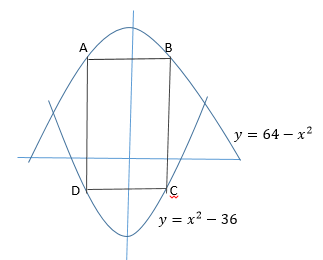### Test: PSAT Math1 Rectangle ABCD is shown in the figure above. Points A and B lie on the graph of y = 64 – x2 , and points C and D lie on the graph of y = x2 – 36. Segments AD and BC are both parallel to the y-axis. The x-coordinates of points A and B are equal to –k and k, respectively. If the value of k changes from 2 to 4, by how much will the area of rectangle ABCD increase?

272

176

544

88

352

1/11 questions

0%

## Access results and powerful study features!

Take 15 seconds to create an account.